# 20 Results

View
Selected filters:
• MI.Math.Content.8.EE.B.6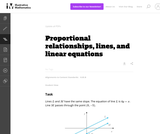Unrestricted Use
CC BY
Rating

This is a task from the Illustrative Mathematics website that is one part of a complete illustration of the standard to which it is aligned. Each task has at least one solution and some commentary that addresses important asects of the task and its potential use. Here are the first few lines of the commentary for this task: Lines \$L\$ and \$M\$ have the same slope. The equation of line \$L\$ is \$4y=x\$. Line \$M\$ passes through the point \$(0, -5)\$. What is the equation of line \$M...

Subject:
Mathematics
Material Type:
Activity/Lab
Provider:
Illustrative Mathematics
Provider Set:
Illustrative Mathematics
Author:
Illustrative Mathematics
05/28/2013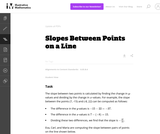Unrestricted Use
CC BY
Rating

This is a task from the Illustrative Mathematics website that is one part of a complete illustration of the standard to which it is aligned. Each task has at least one solution and some commentary that addresses important asects of the task and its potential use. Here are the first few lines of the commentary for this task: The slope between two points is calculated by finding the change in \$y\$-values and dividing by the change in \$x\$-values. For example, the slope between...

Subject:
Mathematics
Material Type:
Activity/Lab
Provider:
Illustrative Mathematics
Provider Set:
Illustrative Mathematics
Author:
Illustrative Mathematics
08/19/2013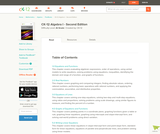Conditional Remix & Share Permitted
CC BY-NC-SA
Rating

A work in progress, CK-12's Algebra I Second Edition is a clear presentation of algebra for the high school student. Topics include: Equations and Functions, Real Numbers, Equations of Lines, Solving Systems of Equations and Quadratic Equations.

Subject:
Algebra
Functions
Material Type:
Textbook
Provider:
CK-12 Foundation
Provider Set:
CK-12 FlexBook
Author:
Andrew
Annamaria
Anne
Eve
Farbizio
Gloag
Rawley
12/03/2010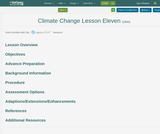Conditional Remix & Share Permitted
CC BY-NC
Rating

Climagraphs can tell us about the seasonal shifts in climate due to climate change. Changes in growing season and water balance in the Great Lakes region will have economic impacts.

Subject:
Environmental Science
Material Type:
Activity/Lab
Lesson Plan
Author:
Michigan Geographic Alliance
08/28/2020Unrestricted Use
CC BY
Rating

This task requires students to work with unit rates.

Subject:
Mathematics
Material Type:
Activity/Lab
Provider:
Illustrative Mathematics
Provider Set:
Illustrative Mathematics
Author:
Illustrative Mathematics
05/01/2012Unrestricted Use
CC BY
Rating

This task requires students to use the fact that on the graph of the linear equation y=ax+c, the y-coordinate increases by a when x increases by one. Specific values for c and d were left out intentionally to encourage students to use the above fact as opposed to computing the point of intersection, (p,q), and then computing respective function values to answer the question.

Subject:
Mathematics
Material Type:
Activity/Lab
Provider:
Illustrative Mathematics
Provider Set:
Illustrative Mathematics
Author:
Illustrative Mathematics
05/01/2012Unrestricted Use
CC BY
Rating

This task helps students solidify their understanding of linear functions and push them to be more fluent in their reasoning about slope and y-intercepts. This task has also produced a reasonable starting place for discussing point-slope form of a linear equation.

Subject:
Mathematics
Material Type:
Activity/Lab
Provider:
Illustrative Mathematics
Provider Set:
Illustrative Mathematics
Author:
Illustrative Mathematics
05/01/2012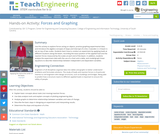Educational Use
Rating

Use this activity to explore forces acting on objects, practice graphing experimental data, and introduce the algebra concepts of slope and intercept of a line. A wooden 2 x 4 beam is set on top of two scales. Students learn how to conduct an experiment by applying loads at different locations along the beam, recording the exact position of the applied load and the reaction forces measured by the scales at each end of the beam. In addition, students analyze the experiment data with the use of a chart and a table, and model/graph linear equations to describe relationships between independent and dependent variables.

Subject:
Engineering
Mathematics
Physics
Material Type:
Activity/Lab
Provider:
TeachEngineering
Provider Set:
TeachEngineering
Author:
Ivanka Todorova
Jed Lyons
09/18/2014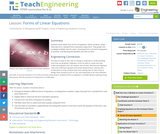Educational Use
Rating

Students learn about four forms of equations: direct variation, slope-intercept form, standard form and point-slope form. They graph and complete problem sets for each, converting from one form of equation to another, and learning the benefits and uses of each.

Subject:
Engineering
Mathematics
Algebra
Material Type:
Lesson Plan
Provider:
TeachEngineering
Provider Set:
TeachEngineering
Author:
Aubrey McKelvey
09/18/2014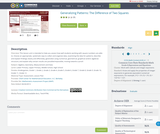Only Sharing Permitted
CC BY-NC-ND
Rating

This lesson unit is intended to help you assess how well students working with square numbers are able to: choose an appropriate, systematic way to collect and organize data, examining the data for patterns; describe and explain findings clearly and effectively; generalize using numerical, geometrical, graphical and/or algebraic structure; and explain why certain results are possible/impossible, moving towards a proof.

Subject:
Algebra
Geometry
Measurement and Data
Material Type:
Assessment
Lesson Plan
Author:
Shell Center for Mathematical Education
U.C. Berkeley
08/10/2020Conditional Remix & Share Permitted
CC BY-NC-SA
Rating

In Module 4, students extend what they already know about unit rates and proportional relationships to linear equations and their graphs.  Students understand the connections between proportional relationships, lines, and linear equations in this module.  Students learn to apply the skills they acquired in Grades 6 and 7, with respect to symbolic notation and properties of equality to transcribe and solve equations in one variable and then in two variables.

Subject:
Mathematics
Material Type:
Module
Author:
EngageNY
New York State Education Department
08/06/2020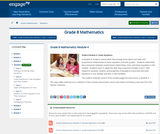Conditional Remix & Share Permitted
CC BY-NC-SA
Rating

In Module 4, students extend what they already know about unit rates and proportional relationships to linear equations and their graphs.  Students understand the connections between proportional relationships, lines, and linear equations in this module.  Students learn to apply the skills they acquired in Grades 6 and 7, with respect to symbolic notation and properties of equality to transcribe and solve equations in one variable and then in two variables.

Subject:
Algebra
Material Type:
Module
Provider:
New York State Education Department
Provider Set:
EngageNY
11/12/2013Conditional Remix & Share Permitted
CC BY-NC-SA
Rating

In this lesson designed to enhance literacy skills, students learn how to read and interpret a distance&ndash;time graph.

Subject:
Mathematics
Material Type:
Activity/Lab
Author:
PBS LearningMedia
08/04/2020Educational Use
Rating

Students learn about an important characteristic of lines: their slopes. Slope can be determined either in graphical or algebraic form. Slope can also be described as positive, negative, zero or undefined. Students get an explanation of when and how these different types of slope occur. Finally, they learn how slope relates to parallel and perpendicular lines. When two lines are parallel, they have the same slope and when they are perpendicular their slopes are negative reciprocals of one another.

Subject:
Engineering
Mathematics
Algebra
Material Type:
Lesson Plan
Provider:
TeachEngineering
Provider Set:
TeachEngineering
Author:
Aubrey Mckelvey
09/18/2014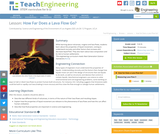Educational Use
Rating

While learning about volcanoes, magma and lava flows, students learn about the properties of liquid movement, coming to understand viscosity and other factors that increase and decrease liquid flow. They also learn about lava composition and its risk to human settlements.

Subject:
Engineering
Mathematics
Physics
Material Type:
Lesson Plan
Provider:
TeachEngineering
Provider Set:
TeachEngineering
Author:
Brittany Enzmann
09/18/2014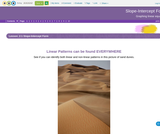Unrestricted Use
CC BY
Rating

This lesson is an introduction to learning to graph linear equations written in Slope-Intercept Form. It was written for the Beginning Algebra 1 student.

Subject:
Algebra
Material Type:
Teaching/Learning Strategy
Author:
Jill Oliver
08/07/2020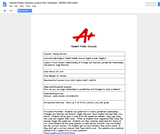Unrestricted Use
CC BY
Rating

The lesson on triangle sum theorem, exterior angle theorem and parallel line relationships revolved around the online teaching tool Quizlet live.  I used this technology as a review of the concepts before they took a quiz as a reinforcement of the material.

Subject:
Mathematics
Geometry
Material Type:
Lesson
Provider:
Michigan Virtual
Author:
Wendy McCann
04/28/2017Only Sharing Permitted
CC BY-NC-ND
Rating

This lesson unit is intended to help teachers assess how well students are able to: translate between decimal and fraction notation, particularly when the decimals are repeating; create and solve simple linear equations to find the fractional equivalent of a repeating decimal; and understand the effect of multiplying a decimal by a power of 10.

Subject:
Mathematics
Material Type:
Assessment
Lesson Plan
Author:
Mathematics Assessment Project (MAP)
Shell Center for Mathematical Education
08/07/2020Only Sharing Permitted
CC BY-NC-ND
Rating

This lesson unit is intended to help teachers assess how well students are able to: interpret a situation and represent the variables mathematically; select appropriate mathematical methods to use; explore the effects of systematically varying the constraints; interpret and evaluate the data generated and identify the break-even point, checking it for confirmation; and communicate their reasoning clearly.

Subject:
Mathematics
Material Type:
Assessment
Lesson Plan
Author:
Mathematics Assessment Project (MAP)
Shell Center for Mathematical Education
08/07/2020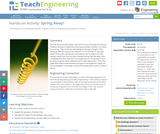Educational Use
Rating

This lab demonstrates Hooke's Law with the use of springs and masses. Students attempt to determine the proportionality constant, or k-value, for a spring. They do this by calculating the change in length of the spring as different masses are added to it. The concept of a spring's elastic limit is also introduced, and the students test to makes sure the spring's elastic limit has not been reached during their lab tests. After compiling their data, they attempt to find an average value of the spring's k-value by measuring the slopes between each of their data points. Then they apply what they've learned about springs to how engineers might use that knowledge in the design of a toy that enables kids to jump 2-3 feet in the air.

Subject:
Engineering
Life Science
Mathematics
Material Type:
Activity/Lab
Provider:
TeachEngineering
Provider Set:
TeachEngineering
Author:
Aubrey Mckelvey